PRINTABLE FOR KIDS

XII (12) HSC

XI (11) FYJC
X (10) SSC

### Practice Set 3.2 Arithmetic Progression Class 10th Mathematics Part 1 MHB Solution

Practice Set 3.2

1, 8, 15, 22, . . . Here a = square , t_1 = square , t_2 = square , t_3 = square…

3, 6, 9, 12, . . . Here t_1 = square , t_2 = square , t_3 = square , t_4 = square…

- 3, - 8, - 13, - 18, . . . Here t_3 = square , t_2 = square , t_4 = square , t_1 =…

70, 60, 50, 40, . . . Here t_1 = square , t_2 = square , t_3 = square therefore a =…

Decide whether following sequence is an A.P., if so find the 20th term of the…

Given Arithmetic Progression 12, 16, 20, 24, . . . Find the 24th term of this…

Find the 19th term of the following A.P. 7, 13, 19, 25, . . .

Find the 27th term of the following A.P. 9, 4, - 1, - 6, - 11, . . .…

Find how many three digit natural numbers are divisible by 5.

The 11th term and the 21st term of an A.P. are 16 and 29 respectively, then find the…

11, 8, 5, 2, . . . In this A.P. which term is number - 151?

In the natural numbers from 10 to 250, how many are divisible by 4?…

In an A.P. 17th term is 7 more than its 10th term. Find the common difference.…

###### Practice Set 3.2

Question 1.

Write the correct number in the given boxes from the following A. P.

1, 8, 15, 22, . . .

Here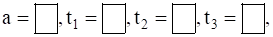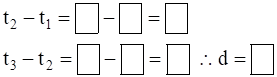1, 8, 15, 22, . . .

First term a = 1

Second term t1 = 8

Third term t2 = 15

Fourth term t3 = 22

We know that d = tn + 1 – tn

Thus, t2 – t1 = 15 – 8 = 7

t3 – t2 = 22 – 15 = 7

Thus, d = 7

Question 2.

Write the correct number in the given boxes from the following A. P.

3, 6, 9, 12, . . .

Here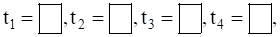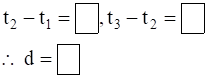3,6,9,12, . . .

First term a = 3

Second term t1 = 6

Third term t2 = 9

Fourth term t3 = 12

We know that d = tn + 1 – tn

Thus, t2 – t1 = 9 – 6 = 3

t3 – t2 = 12 – 9 = 3

Thus, d = 3

Question 3.

Write the correct number in the given boxes from the following A. P.

– 3, – 8, – 13, – 18, . . .

Here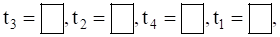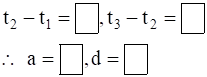– 3, – 8, – 13, – 18, . . .

First term a = – 3

Second term t1 = – 8

Third term t2 = – 13

Fourth term t3 = – 18

We know that d = tn + 1 – tn

Thus, t2 – t1 = – 13 – ( – 8) = – 13 + 8 = – 5

t3 – t2 = – 18 – ( – 13) = – 18 + 13 = – 5

Thus, d = – 5

Question 4.

Write the correct number in the given boxes from the following A. P.

70, 60, 50, 40, . . .

Here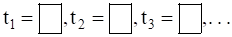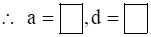70, 60, 50, 40, . . .

First term a = 70

Second term t1 = 60

Third term t2 = 50

Fourth term t3 = 40

We know that d = tn + 1 – tn

Thus, t2 – t1 = 50 – 60 = – 10

t3 – t2 = 40 – 50 = – 10

Thus, d = – 10

Question 5.

Decide whether following sequence is an A.P., if so find the 20th term of the progression.

– 12, – 5, 2, 9, 16, 23, 30, . . .

Given A.P. is – 12, – 5, 2, 9, 16, 23, 30, . . .

Where first term a = – 12

Second term t1 = – 5

Third term t2 = 2

Common Difference d = t2 – t1 = 2 – ( – 5) = 2 + 5 = 7

We know that, nth term of an A.P. is

tn = a + (n – 1)d

We need to find the 20th term,

Here n = 20

Thus, t20 = – 12 + (20 – 1)× 7

t20 = – 12 + (19)× 7 = – 12 + 133 = 121

Thus, t20 = 121

Question 6.

Given Arithmetic Progression 12, 16, 20, 24, . . . Find the 24th term of this progression.

Given A.P. is 12, 16, 20, 24, . . .

Where first term a = 12

Second term t1 = 16

Third term t2 = 20

Common Difference d = t2 – t1 = 20 – 16 = 4

We know that, nth term of an A.P. is

tn = a + (n – 1)d

We need to find the 24th term,

Here n = 24

Thus, t24 = 12 + (24 – 1)× 4

t24 = 12 + (23)× 4 = 12 + 92 = 104

Thus, t24 = 104

Question 7.

Find the 19th term of the following A.P.

7, 13, 19, 25, . . .

Given A.P. is 7, 13, 19, 25, . . .

Where first term a = 7

Second term t1 = 13

Third term t2 = 19

Common Difference d = t2 – t1 = 19 – 13 = 6

We know that, nth term of an A.P. is

tn = a + (n – 1)d

We need to find the 19th term,

Here n = 19

Thus, t19 = 7 + (19 – 1)× 6

t19 = 7 + (18)× 6 = 7 + 108 = 115

Thus, t19 = 115

Question 8.

Find the 27th term of the following A.P.

9, 4, – 1, – 6, – 11, . . .

Given A.P. is 9, 4, – 1, – 6, – 11, . . .

Where first term a = 9

Second term t1 = 4

Third term t2 = – 1

Common Difference d = t2 – t1 = – 1 – 4 = – 5

We know that, nth term of an A.P. is

tn = a + (n – 1)d

We need to find the 27th term,

Here n = 27

Thus, t27 = 9 + (27 – 1)× ( – 5)

t27 = 9 + (26)× ( – 5) = 9 – 130 = – 121

Thus, t27 = – 121

Question 9.

Find how many three digit natural numbers are divisible by 5.

List of three digit number divisible by 5 are

100, 105,110,115,……….. 995

Let us find how many such number are there?

From the above sequence, we know that

tn = 995, a = 100

t1 = 105, t2 = 110

Thus, d = t2 – t1 = 110 – 105 = 5

Now, By using nth term of an A.P. formula

tn = a + (n – 1)d

we can find value of “n”

Thus, on substituting all the value in formula we get,

995 = 100 + (n – 1)× 5

⇒ 995 – 100 = (n – 1)× 5

⇒ 895 = (n – 1) × 5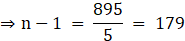⇒ n = 179 + 1 = 180

Question 10.

The 11th term and the 21st term of an A.P. are 16 and 29 respectively, then find the 41th term of that A.P.

Given: t11 = 16 and t21 = 29

To find: t41

Using nth term of an A.P. formula

tn = a + (n – 1)d

we will find value of “a” and “d”

Let, t11 = a + (11 – 1) d

⇒ 16 = a + 10 d …..(1)

t21 = a + (21 – 1) d

⇒ 29 = a + 20 d …..(2)

Subtracting eq. (1) from eq. (2), we get,

⇒ 29 – 16 = (a – a) + (20 d – 10 d)

⇒ 13 = 10 d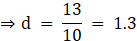Substitute value of “d” in eq. (1) to get value of “a”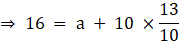⇒ 16 = a + 13

⇒ a = 16 – 13 = 3

Now, we will find the value of t41 using nth term of an A.P. formula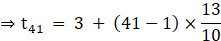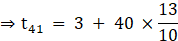⇒ t41 = 3 + 4 × 13 = 3 + 52 = 55

Thus, t41 = 55

Question 11.

11, 8, 5, 2, . . . In this A.P. which term is number – 151?

By, given A.P. 11, 8, 5, 2, . . .

we know that

a = 11, t1 = 8, t2 = 5

Thus, d = t2 – t1 = 5 – 8 = – 3

Given: tn = – 151

Now, By using nth term of an A.P. formula

tn = a + (n – 1)d

we can find value of “n”

Thus, on substituting all the value in formula we get,

– 151 = 11 + (n – 1)× ( – 3)

⇒ – 151 – 11 = (n – 1)× ( – 3)

⇒ – 162 = (n – 1) × ( – 3)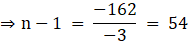⇒ n = 54 + 1 = 55

Question 12.

In the natural numbers from 10 to 250, how many are divisible by 4?

List of number divisible by 4 in between 10 to 250 are

12, 16,20,24,……….. 248

Let us find how many such number are there?

From the above sequence, we know that

tn = 248, a = 12

t1 = 16, t2 = 20

Thus, d = t2 – t1 = 20 – 16 = 4

Now, By using nth term of an A.P. formula

tn = a + (n – 1)d

we can find value of “n”

Thus, on substituting all the value in formula we get,

248 = 12 + (n – 1)× 4

⇒ 248 – 12 = (n – 1)× 4

⇒ 236 = (n – 1) × 4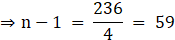⇒ n = 59 + 1 = 60

Question 13.

In an A.P. 17th term is 7 more than its 10th term. Find the common difference.

Given: t17 = 7 + t10 ……(1)

In t17, n = 17

In t10, n = 10

By using nth term of an A.P. formula,

tn = a + (n – 1)d

where n = no. of terms

a = first term

d = common difference

tn = nth term

Thus, on using formula in eq. (1) we get,

⇒ a + (17 – 1)d = 7 + (a + (10 – 1)d)

⇒ a + 16 d = 7 + (a + 9 d)

⇒ a + 16 d – a – 9 d = 7

⇒ 7 d = 7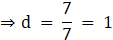Thus, common difference “d” = 1

## PDF FILE TO YOUR EMAIL IMMEDIATELY PURCHASE NOTES & PAPER SOLUTION. @ Rs. 50/- each (GST extra)

SUBJECTS

HINDI ENTIRE PAPER SOLUTION

MARATHI PAPER SOLUTION
SSC MATHS I PAPER SOLUTION
SSC MATHS II PAPER SOLUTION
SSC SCIENCE I PAPER SOLUTION
SSC SCIENCE II PAPER SOLUTION
SSC ENGLISH PAPER SOLUTION
SSC & HSC ENGLISH WRITING SKILL
HSC ACCOUNTS NOTES
HSC OCM NOTES
HSC ECONOMICS NOTES
HSC SECRETARIAL PRACTICE NOTES

2019 Board Paper Solution

HSC ENGLISH SET A 2019 21st February, 2019

HSC ENGLISH SET B 2019 21st February, 2019

HSC ENGLISH SET C 2019 21st February, 2019

HSC ENGLISH SET D 2019 21st February, 2019

SECRETARIAL PRACTICE (S.P) 2019 25th February, 2019

HSC XII PHYSICS 2019 25th February, 2019

CHEMISTRY XII HSC SOLUTION 27th, February, 2019

OCM PAPER SOLUTION 2019 27th, February, 2019

HSC MATHS PAPER SOLUTION COMMERCE, 2nd March, 2019

HSC MATHS PAPER SOLUTION SCIENCE 2nd, March, 2019

SSC ENGLISH STD 10 5TH MARCH, 2019.

HSC XII ACCOUNTS 2019 6th March, 2019

HSC XII BIOLOGY 2019 6TH March, 2019

HSC XII ECONOMICS 9Th March 2019

SSC Maths I March 2019 Solution 10th Standard11th, March, 2019

SSC MATHS II MARCH 2019 SOLUTION 10TH STD.13th March, 2019

SSC SCIENCE I MARCH 2019 SOLUTION 10TH STD. 15th March, 2019.

SSC SCIENCE II MARCH 2019 SOLUTION 10TH STD. 18th March, 2019.

SSC SOCIAL SCIENCE I MARCH 2019 SOLUTION20th March, 2019

SSC SOCIAL SCIENCE II MARCH 2019 SOLUTION, 22nd March, 2019

XII CBSE - BOARD - MARCH - 2019 ENGLISH - QP + SOLUTIONS, 2nd March, 2019

HSC Maharashtra Board Papers 2020

(Std 12th English Medium)

HSC ECONOMICS MARCH 2020

HSC OCM MARCH 2020

HSC ACCOUNTS MARCH 2020

HSC S.P. MARCH 2020

HSC ENGLISH MARCH 2020

HSC HINDI MARCH 2020

HSC MARATHI MARCH 2020

HSC MATHS MARCH 2020

SSC Maharashtra Board Papers 2020

(Std 10th English Medium)

English MARCH 2020

HindI MARCH 2020

Hindi (Composite) MARCH 2020

Marathi MARCH 2020

Mathematics (Paper 1) MARCH 2020

Mathematics (Paper 2) MARCH 2020

Sanskrit MARCH 2020

Important-formula

THANKS